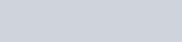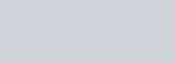In the next tutorial I introduce you to the cumulative distribution function of a probability density function. All very confusing maybe but hopefully not after watching the next video. In it you will be shown how to do the following question.

Giveni) Sketch the p.d.f. f(x)
ii) Find F(1)
iii) Find F(x)

Cumulative Distribution Functions : Introduction : ExamSolutions - youtube Video

Example

I have picked this next example as it has two functions in the p.d.f. and finding the c.d.f. can cause problems. Try it and check your answer against mine.

A continuous random variable X has a p.d.f. f(x), defined byFind the cumulative distribution function, F(x)

Cumulative Distribution Function : Example : ExamSolutions - youtube Video